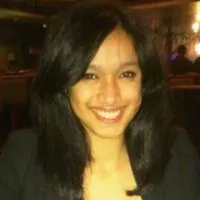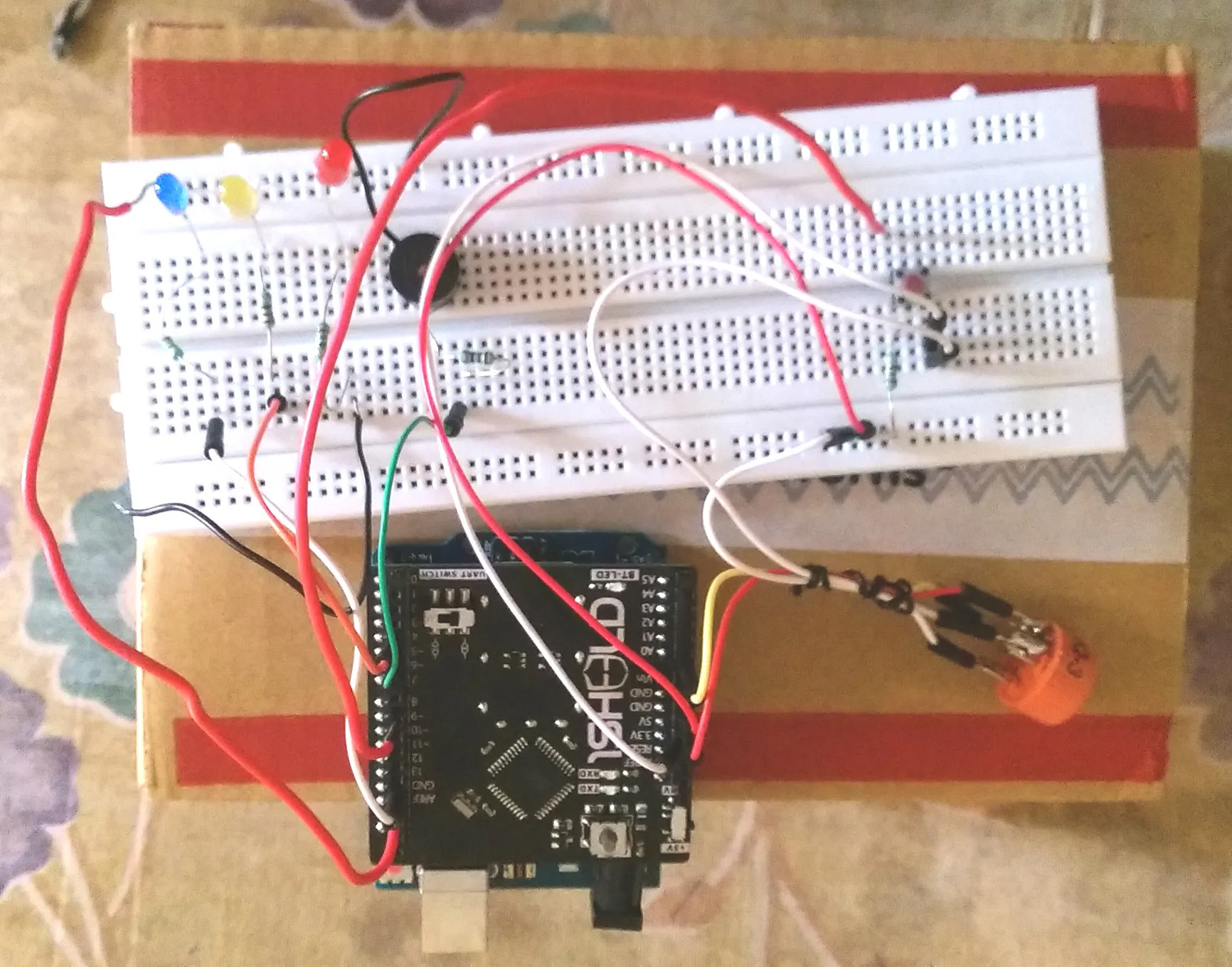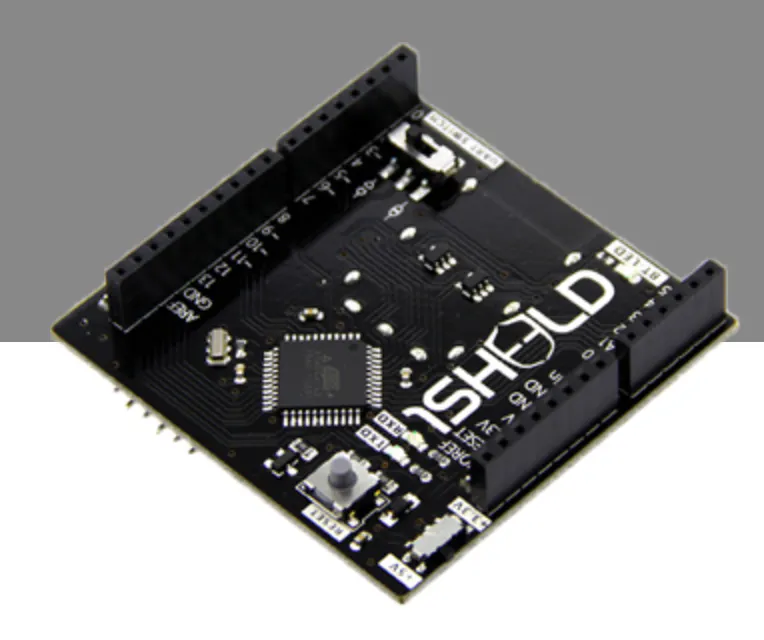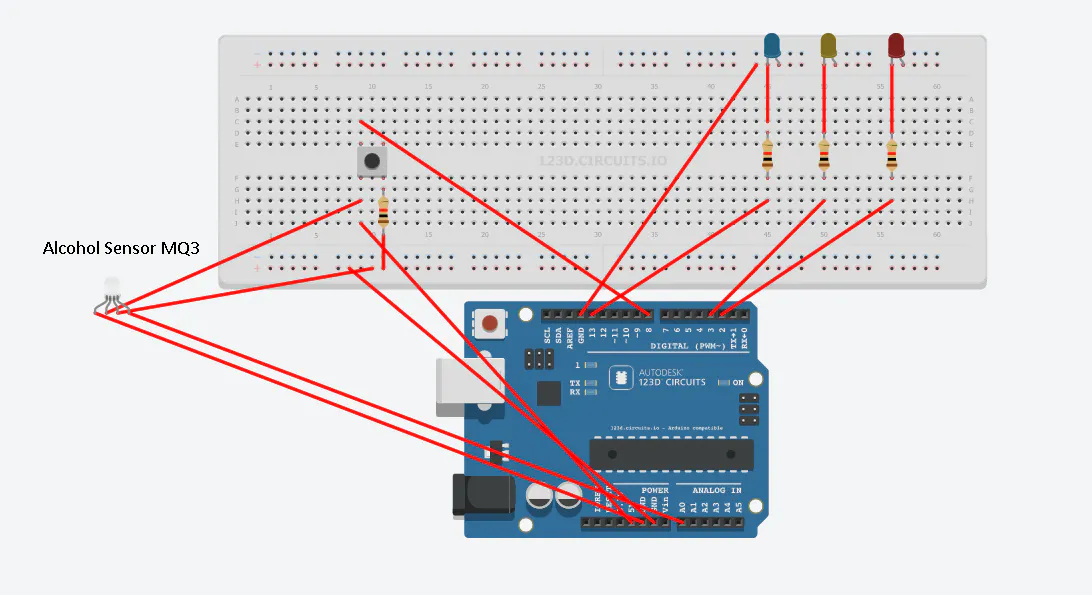# Cab Safety System (Cabs4Cabs)

Cabs4Cabs: Consolidated Arduino Based Sureveillance for Cabs.

BeginnerWork in progress4,982## Things used in this project

### Hardware componentsArduino UNO & Genuino UNO
×11Sheeld
×1
 MQ3 Alcohol Sensor
×1
 Push Button
×1
 Resistors and LEDs
×1

## Schematics## Code

### Arduino Side Code

C/C++
The 1sheeld along with the Arduino Communicated with the ThingSpeak
```#include <OneSheeld.h>

HttpRequest request ("https://api.thingspeak.com/update");
char latc, lonc, sosMessage, temp3, distancec;
float lon=0,lat=0,x=0,y=0,z=0,temp1=0, temp2=0;
int buttonPin = 8, startFlag=3;
bool isMessageSent, sosFlag=false, mishapFlag=false;
int sensorPin = 0;
int time, flag=0;
int i;
int j;
float start_lat=0, start_lon=0, distance=0;

void setup()
{

pinMode(2,OUTPUT); //Initializing pins for LED
pinMode(3,OUTPUT);
pinMode(13,OUTPUT);
pinMode(4,OUTPUT);
pinMode(buttonPin,INPUT); //Intitializing pin for SOS

OneSheeld.begin();

}

void loop()
{

///****ALCOHOL SENSOR****/
Terminal.println(sensor);
if(sensor <= 10 && sensor >=1) //Low Value
{

digitalWrite(2, LOW);
digitalWrite(3, LOW);
digitalWrite(13, HIGH);
digitalWrite(4, LOW);

}
else if(sensor > 10 && sensor < 40) //Moderate Value
{
digitalWrite(2, LOW);
digitalWrite(3, HIGH);
digitalWrite(13, LOW);
digitalWrite(4, LOW);

}
else if(sensor > 40) //High Value
{

digitalWrite(2, HIGH);
digitalWrite(3,HIGH);
digitalWrite(13,HIGH);
digitalWrite(4, HIGH);
}
else if(sensor == 0) //Default Value
{
digitalWrite(2, LOW);
digitalWrite(3, LOW);
digitalWrite(13, LOW);
digitalWrite(4, LOW);

}

/****GPS****/
if ((start_lat==0) && (start_lon==0) && (flag==0)) //Start Location Coordinates
{
start_lat = GPS.getLatitude();
start_lon = GPS.getLongitude();
if ((start_lat!=0) && (start_lon!=0))
{
flag=1;
}
}
lat = GPS.getLatitude(); //Current Location Coordinates
lon = GPS.getLongitude();
dtostrf(lat, 3, 6, latc);
dtostrf(lon, 3, 6, lonc);
distance = GPS.getDistance(start_lat,start_lon);
distance/= 1000;
dtostrf(distance, 3, 3, distancec);
request.addParameter("field5",distancec); //Distance between Start and Current Location

/****MISHAP****/
x=AccelerometerSensor.getX();
y=AccelerometerSensor.getY();
z=AccelerometerSensor.getZ();

if ((x>-7.0) && (z<-7.0)) //Mishap Detected
{
//mishapFlag==true;
}
else
{
}

/****SOS****/
if(digitalRead(buttonPin) == HIGH) //SOS Button Pressed
{
if(!isMessageSent)
{
lat = GPS.getLatitude();
lon = GPS.getLongitude();
sosFlag=true;
dtostrf(lat, 8, 6, latc);
strcat (sosMessage,latc);
strcat(sosMessage,",");
dtostrf(lon, 8, 6, lonc);
strcat (sosMessage,lonc);
SMS.send("+91xxxxxxxxx",sosMessage); //SMS to emergency contact
isMessageSent = true;
}

else
{
isMessageSent = false;
}
}
else
{
if(sosFlag==false)
{
}
}

Internet.performGet(request);
OneSheeld.delay(5000);

}
```